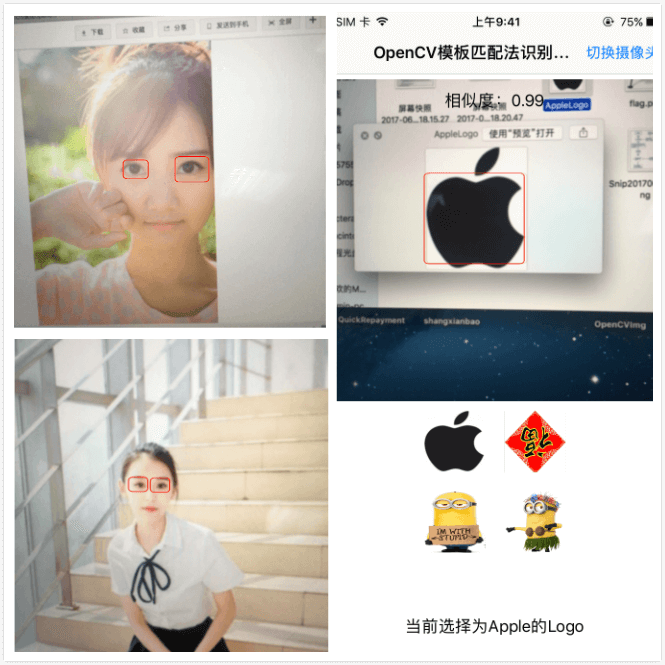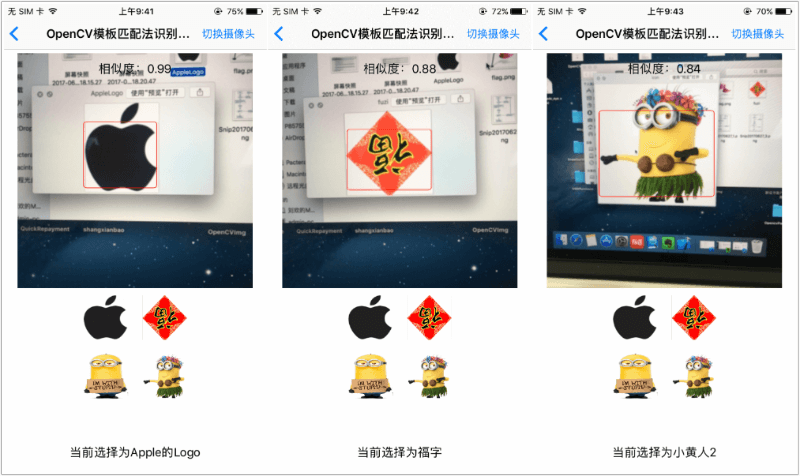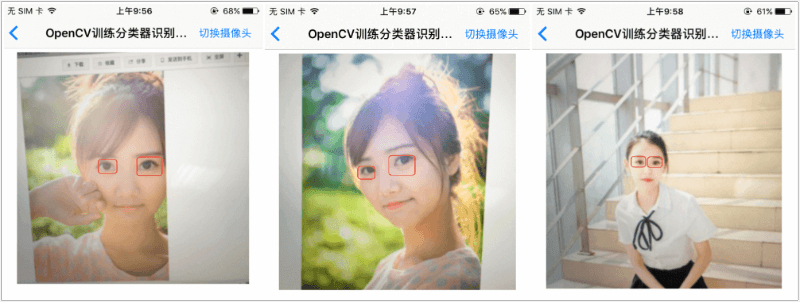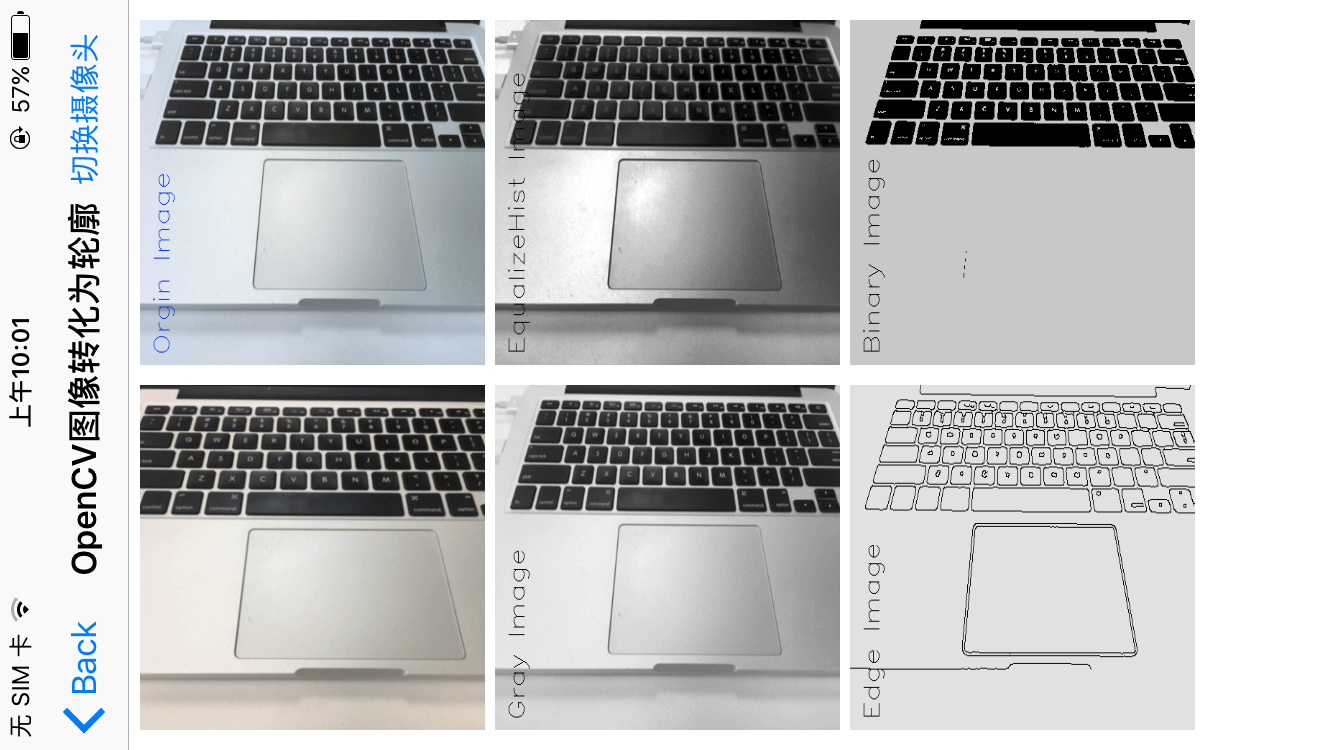## 前言

1. 基于规则运算的图像识别，例如颜色形状等特征匹配。
2. 基于统计的图像识别，例如机器学习自动提取特征，并通过级联多特征匹配。
3. 场景：特征匹配方法适合固定的场景或物体识别，机器学习方法适合大量具有共同特征的场景或物体识别。
4. 优劣：无论从识别率，准确度，还是适应多变场景来讲，机器学习都是优于特征匹配方法的，前提你有`大量的数据`来训练分类器。如果是仅仅是识别特定场景、物体或者形状，使用模板匹配方法更简单更易于实现。## 方案选择

iOS 客户端快速实现图像识别的两种方案:

TensorFlow Google AlphaGo 战胜世界围棋冠军，人工智能大火，谷歌 2016 年开源了其用来制作 AlphaGo 的深度学习系统 Tensorflow，而且 Tensorflow 支持了 iOS，Android 等移动端。
OpenCV Intel OpenCV 于 1999 年由 Intel 建立的，跨平台的开源计算机视觉库，主要由 C 和 C++ 代码构成，有 Python、Ruby、MATLAB 等语言的接口，支持 iOS，Android 等移动设备。

TensorFlow && OpenCV## OpenCV 中常用图像识别的方法对比

2. 适用于某个图片是另外一张大图的一部分的场景；
3. 例如五角星形状固定，可转换为边框匹配。

2. 例如全景图片的拼接，长图的拼接；
3. 监控视频中的目标跟踪。

2. 支付宝扫福，福字有成千上万种写法。

## 集成 OpenCV

iOS项目集成OpenCV，主要有两种方法：

1. OpenCV官网下载 opencv2.framework 框架，拖入即可，导入依赖的库，具体集成方法见我的另外一篇文章iOS集成OpenCV

2. CocoaPods 方式集成，Pod 文件中配置 `pod 'OpenCV'`，此方法简单，推荐。

## 模板匹配法

``````#pragma mark - 获取视频帧，处理视频
- (void)captureOutput:(AVCaptureOutput *)captureOutput didOutputSampleBuffer:(CMSampleBufferRef)sampleBuffer fromConnection:(AVCaptureConnection *)connection;
``````

``````/**
* 高效将视频流转换为 Mat 图像矩阵
* Efficiently convert video streams to Mat image matrices
@param sampleBuffer 视频流(video stream)
@return OpenCV 可用的图像矩阵(OpenCV available image matrix)
*/
+(cv::Mat)bufferToGrayMat:(CMSampleBufferRef) sampleBuffer{
CVPixelBufferRef pixelBuffer = CMSampleBufferGetImageBuffer(sampleBuffer);
OSType format = CVPixelBufferGetPixelFormatType(pixelBuffer);
if (format != kCVPixelFormatType_420YpCbCr8BiPlanarFullRange) {
OOBLog(@"Only YUV is supported"); // Y 是亮度，UV 是颜色
return cv::Mat();
}
CVPixelBufferLockBaseAddress(pixelBuffer, 0);
void *baseaddress = CVPixelBufferGetBaseAddressOfPlane(pixelBuffer, 0);
CGFloat width = CVPixelBufferGetWidth(pixelBuffer);
videoRenderWidth = width; // 保存渲染宽度
CGFloat colCount = CVPixelBufferGetBytesPerRowOfPlane(pixelBuffer, 0);
if (width != colCount) {
width = colCount; // 如果有字节对齐
}
CGFloat height = CVPixelBufferGetHeight(pixelBuffer);
cv::Mat mat(height, width, CV_8UC1, baseaddress, 0);
CVPixelBufferUnlockBaseAddress(pixelBuffer, 0);
return mat;
}

``````

``````///MARK: - 将CMSampleBufferRef转为cv::Mat
+(cv::Mat)bufferToMat:(CMSampleBufferRef) sampleBuffer{
CVImageBufferRef imgBuf = CMSampleBufferGetImageBuffer(sampleBuffer);
//锁定内存
CVPixelBufferLockBaseAddress(imgBuf, 0);
// get the address to the image data
void *imgBufAddr = CVPixelBufferGetBaseAddress(imgBuf);
// get image properties
int w = (int)CVPixelBufferGetWidth(imgBuf);
int h = (int)CVPixelBufferGetHeight(imgBuf);
// create the cv mat
cv::Mat mat(h, w, CV_8UC4, imgBufAddr, 0);
//转换为灰度图像
cv::Mat edges;
cv::cvtColor(mat, edges, CV_BGR2GRAY);
//旋转90度
cv::Mat transMat;
cv::transpose(mat, transMat);
//翻转,1是x方向，0是y方向，-1位Both
cv::Mat flipMat;
cv::flip(transMat, flipMat, 1);
CVPixelBufferUnlockBaseAddress(imgBuf, 0);
return flipMat;
}

``````

``````/**
对比两个图像是否有相同区域
@return 有为Yes
*/
-(BOOL)compareInput:(cv::Mat) inputMat templateMat:(cv::Mat)tmpMat{
int result_rows = inputMat.rows - tmpMat.rows + 1;
int result_cols = inputMat.cols - tmpMat.cols + 1;
cv::Mat resultMat = cv::Mat(result_cols,result_rows,CV_32FC1);
cv::matchTemplate(inputMat, tmpMat, resultMat, cv::TM_CCOEFF_NORMED);
double minVal, maxVal;
cv::Point minLoc, maxLoc, matchLoc;
cv::minMaxLoc( resultMat, &minVal, &maxVal, &minLoc, &maxLoc, cv::Mat());
//    matchLoc = maxLoc;
//    NSLog(@"min==%f,max==%f",minVal,maxVal);
dispatch_async(dispatch_get_main_queue(), ^{
self.similarLevelLabel.text = [NSString stringWithFormat:@"相似度：%.2f",maxVal];
});
if (maxVal > 0.7) {
//有相似位置，返回相似位置的第一个点
currentLoc = maxLoc;
return YES;
}else{
return NO;
}
}
``````

## 模板匹配法优化

``````/**
* 对比两个图像是否有相同区域
* Compare whether two images have the same area
@param inputMat 缩放后的视频图像矩阵(Scaled video image matrix)
@param tmpMat 待识别的目标图像矩阵(Target image matrix to be identified)
@param scale 视频缩放比例(video scaling)
@param similarValue 设置的对比相似度阈值(set contrast similarity threshold)
@param videoFillWidth 视频图像字节补齐宽度(Video image byte fill width)
@return 对比结果，包含目标坐标，相似度(comparison result, including target coordinates, similarity)
*/
+(NSDictionary *)compareInput:(Mat) inputMat templateMat:(Mat)tmpMat VideoScale:(CGFloat)scale SimilarValue:(CGFloat)similarValue VideoFillWidth:(CGFloat)videoFillWidth{
// 将待比较的图像缩放至视频宽度的 20% 至 50%
NSArray *tmpArray = @[@(0.2),@(0.3),@(0.4),@(0.5)];
int currentTmpWidth = 0; // 匹配的模板图像宽度
int currentTmpHeight = 0; // 匹配的模板图像高度
double maxVal = 0; // 相似度
cv::Point maxLoc; // 匹配的位置
for (NSNumber *tmpNum in tmpArray) {
CGFloat tmpScale = tmpNum.floatValue;
// 待比较图像宽度，将待比较图像宽度缩放至视频图像的一半左右
int tmpCols = inputMat.cols * tmpScale;
// 待比较图像高度，保持宽高比
int tmpRows = (tmpCols * tmpMat.rows) / tmpMat.cols;
// 缩放后的图像
Mat tmpReMat;
cv::Size tmpReSize = cv::Size(tmpCols,tmpRows);
resize(tmpMat, tmpReMat, tmpReSize);
// 比较结果
int result_rows = inputMat.rows - tmpReMat.rows + 1;
int result_cols = inputMat.cols - tmpReMat.cols + 1;
if (result_rows < 0 || result_cols < 0) {
break;
}
Mat resultMat = Mat(result_cols,result_rows,CV_32FC1);
matchTemplate(inputMat, tmpReMat, resultMat, TM_CCOEFF_NORMED);

double minVal_temp, maxVal_temp;
cv::Point minLoc_temp, maxLoc_temp, matchLoc_temp;
minMaxLoc( resultMat, &minVal_temp, &maxVal_temp, &minLoc_temp, &maxLoc_temp, Mat());
maxVal = maxVal_temp;
if (maxVal >= similarValue) {
maxLoc = maxLoc_temp;
currentTmpWidth = tmpCols;
currentTmpHeight = tmpRows;
break;
}
}

if (maxVal >= similarValue) {
// 目标图像按照缩放比例恢复
CGFloat zoomScale = 1.0 / scale;
CGRect rectF = CGRectMake(maxLoc.x * zoomScale, maxLoc.y * zoomScale, currentTmpWidth * zoomScale, currentTmpHeight * zoomScale);
NSDictionary *tempDict = @{kTargetRect:NSStringFromCGRect(rectF),
kSimilarValue:@(maxVal),
kVideoFillWidth:@(videoFillWidth)};
return tempDict;
}else{
NSDictionary *tempDict = @{kTargetRect:NSStringFromCGRect(CGRectZero),
kSimilarValue:@(maxVal),
kVideoFillWidth:@(videoFillWidth)};
return tempDict;
}
}

``````

## 模板匹配法实现效果图## 加载训练完成的分类器

``````cv::CascadeClassifier icon_cascade;//分类器
``````

``````    //加载训练文件
NSString *bundlePath = [[NSBundle mainBundle] pathForResource:@"haarcascade_eye_tree_eyeglasses.xml" ofType:nil];
cv::String fileName = [bundlePath cStringUsingEncoding:NSUTF8StringEncoding];

BOOL isSuccessLoadFile = icon_cascade.load(fileName);
isSuccessLoadXml = isSuccessLoadFile;
if (isSuccessLoadFile) {
NSLog(@"Load success.......");
}else{
NSLog(@"Load failed......");
}
``````

## 使用分类器识别图像

``````//获取计算出的标记的位置，保存在数组中
-(NSArray *)getTagRectInLayer:(cv::Mat) inputMat{
if (inputMat.empty()) {
return nil;
}
//图像均衡化
cv::equalizeHist(inputMat, inputMat);
//定义向量，存储识别出的位置
std::vector<cv::Rect> glassess;
//分类器识别
icon_cascade.detectMultiScale(inputMat, glassess, 1.1, 3, 0);
//转换为Frame，保存在数组中
NSMutableArray *marr = [NSMutableArray arrayWithCapacity:glassess.size()];
for (NSInteger i = 0; i < glassess.size(); i++) {
CGRect rect = CGRectMake(glassess[i].x, glassess[i].y, glassess[i].width,glassess[i].height);
NSValue *value = [NSValue valueWithCGRect:rect];
[marr addObject:value];
}
return marr.copy;
}
``````## OpenCV 其他图像处理

1. 原图像
2. 直方图均衡化
3. 图像二值化
4. 摄像头预览
5. 灰度图
6. 轮廓图